#### Everything about Standard Error in Excel

In an Excel tutorial, you will learn how to calculate standard error and insert standard error into charts.

The standard error formula is easy to calculate. The only problem is that there is no dedicated Excel function to calculate standard error. Therefore, you need this article to learn how to calculate the standard error step by step and what data you need.

## Definition of standard error

Standard error is one of the important statistical indicators. You can use this indicator to determine the heterogeneity of a sample. This is also very important in forecasting.

There are doubts about the accuracy of the statistics – even when following procedures and using efficient testing equipment. There are statistical formulas in Excel that we can use to calculate the uncertainty. The standard error will help.

## Standard error example

The idea of standard error is very theoretical. To better understand this measure, see the parameter estimation theory. To better illustrate the phenomenon described, let’s look at an example.

Let’s say you want to measure what is the average height of all employees in your company (population ). To obtain the exact value of the average height, you would have to measure all employees. From a practical point of view, it is impossible and unprofitable in large companies.

You have decided to only measure the height of the people on your team (sample). Is this the actual average height of the company as a whole? Most likely not! May be close to the actual value, but most likely not the same.

The mean of the sample (your team) is an estimator (approximation) of the real value in the population (an entire company). If you decided to measure the average height in other teams you would have receive an average score for each test (for each study). Each time, this result would be an “approximation” of the real average height.

A standard error is a measure of the variation of these averages from measures in teams. The more measurements you take, the more accurate your result will be. Standard error will also be reduced.

## Standard Error calculations

To calculate Standard Error you need to calculate the standard deviation first and to know the total number of samples. You can calculate standard error step by step or to use the Excel standard error formula.

Standard Error = Standard deviation / square root of total number of samples

=STDEV(range)/SQRT(COUNT(range))

From the theory and from the equation you may be sure that standard error of the mean is always lower than the standard deviation.

Let’s take a real example. I have three datasets. One for each try. I prepared the data and put the values in a table. This is the link to the learning materials for you to follow.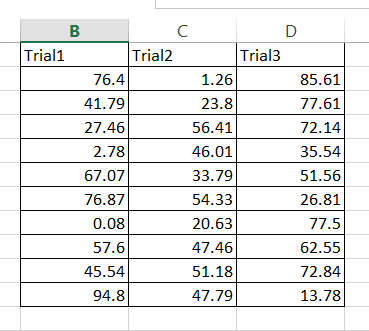### Calculate Standard Error step by step

First to calculate standard error you need to calculate standard deviation. I used =STDEV.S(B2:B11) Excel formula.

Then you need to know the number of samples. In my basic example there are 3 trials per 10 values each.

The final standard error formula is =B13/SQRT(10)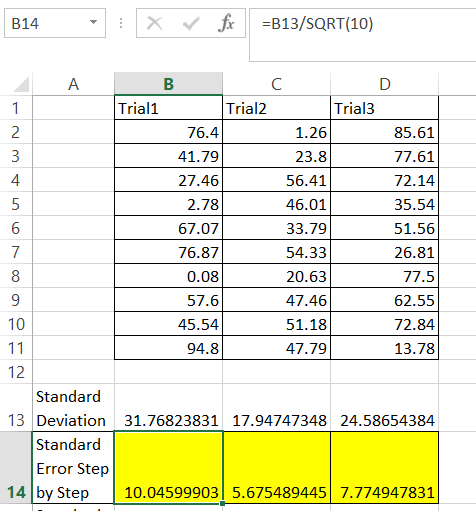### Calculate Standard Error using formula

However knowing the formula you are able to calculate the same using just one single formula.

=STDEV.S(B2:B11)/SQRT(COUNT(B2:B11))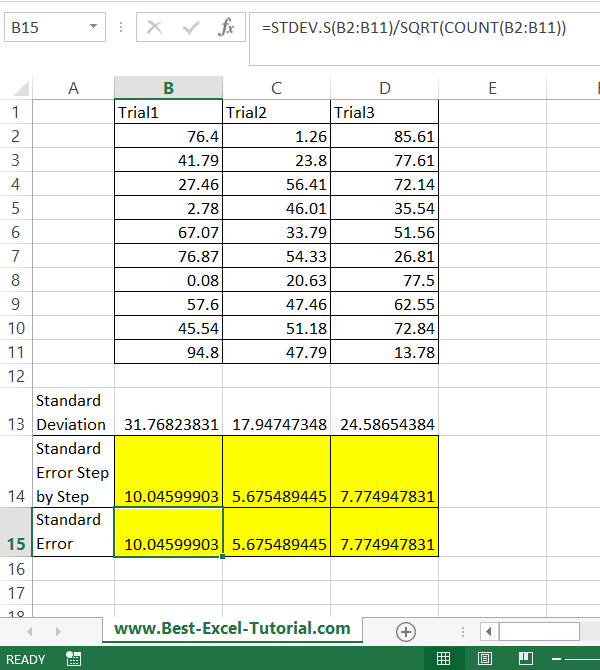The result is the same.

### Calculate Standard Error using Data Analysis

The another method is to use Dana Analysis Toolpak installed. This method you can use to get the whole set of statistics data (standard error included).

First you need to make sure your Data Analysis Toolpak Add-In is installed.

`Here is the lesson how to install Data Analysis Toolpak Add-In.`

Go to Ribbon to the Data tab. Click the Data Analysis button.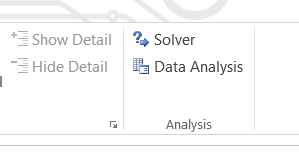New window appears. Choose Descriptive Statistics and click OK.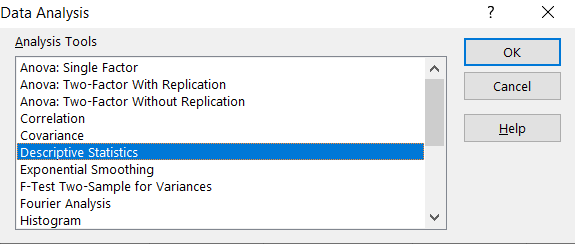Enter the proper parameters:

• Input Range – range of your data (\$B\$1:\$D\$11 in my example)
• Labels in first row – there are labels in my data table
• Output Range – choose where you want to get your standard error calculated (I chose the same Sheet \$G\$1:\$L\$15)
• Summary statistics – make sure it is checked

And this is how to see my Statistics summary. Standard Error you can find in row 4.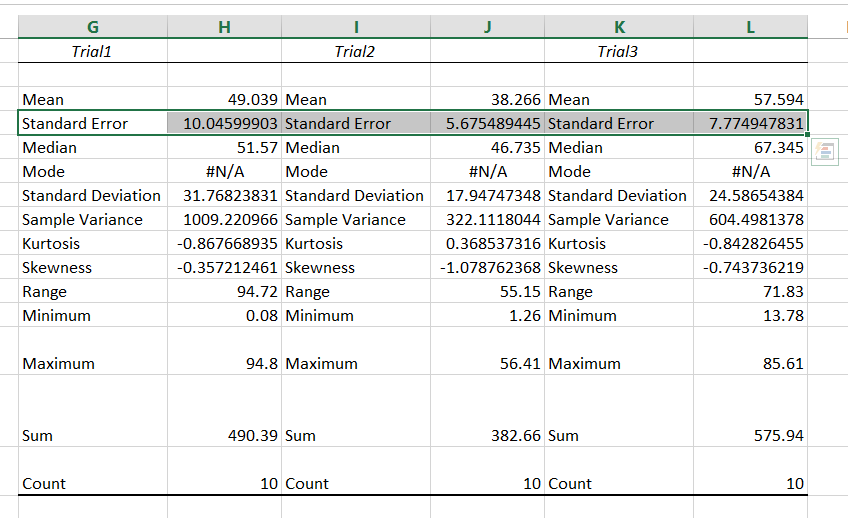## How to show Standard Error of the Mean (SEM) in the Chart?

You may want to show the Standard Error of the mean in the chart. Let’s see how to do that.

To get SEM first calculate mean for each trial. Use Excel average function. The formula is =AVERAGE(B2:B11)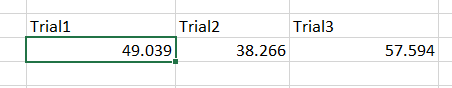Then insert the column chart. Just highlight the data, go to the Ribbon to the Insert tab and choose column chart. The basic chart is visible.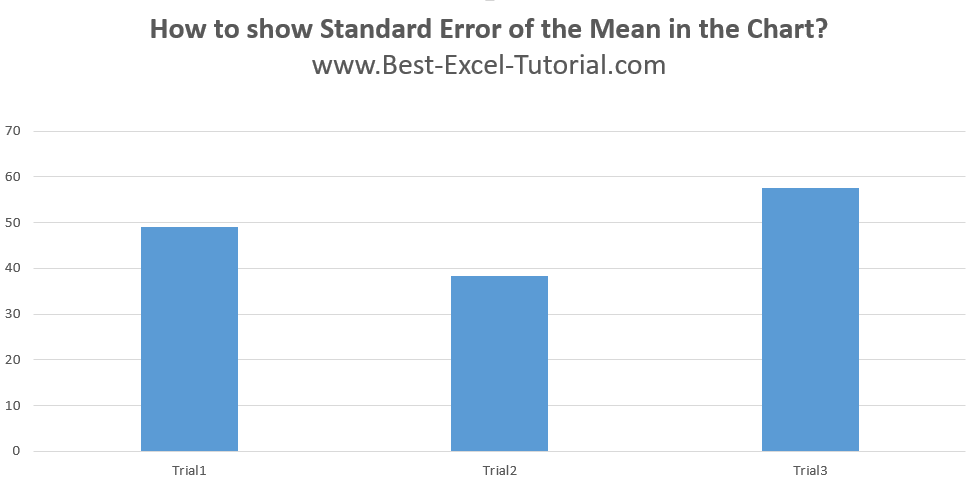This was easy. The more difficult part is to insert sem. To do that click the plus sign on the right side of your chart. Next choose error bars and more options.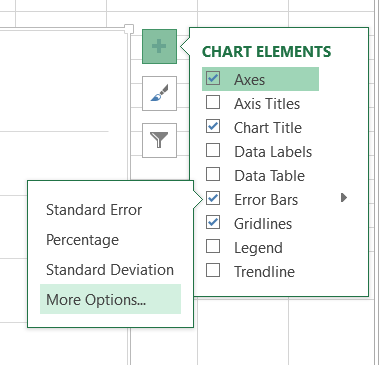In the dialogue choose Custom and click Specify Value.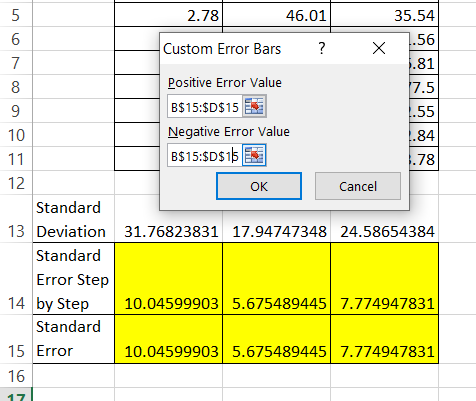For both Positive Error Value and Negative Error Value highlight your calculated Standard Error values.

Now you can see Standard Error lines in the chart.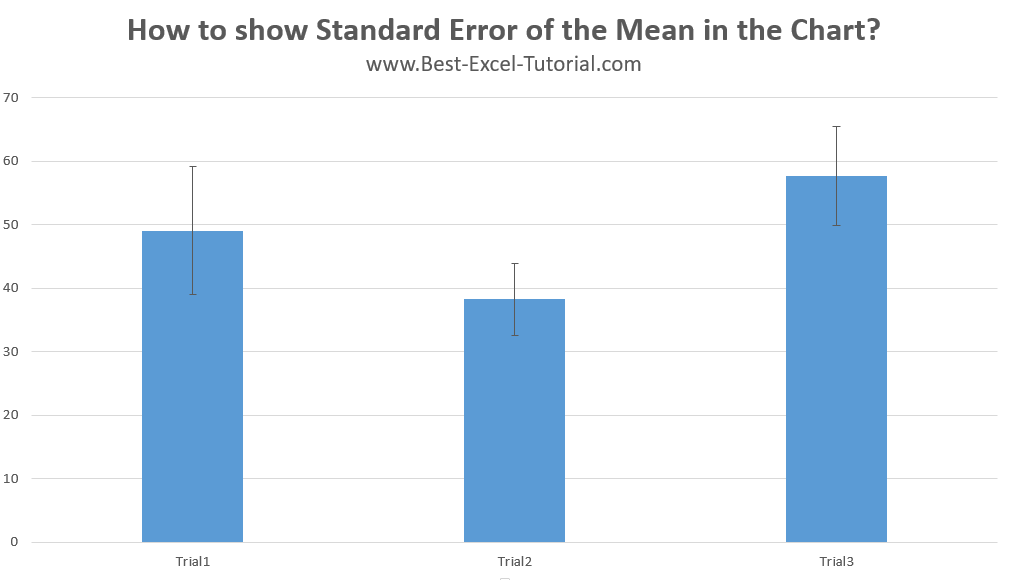It is showing additional information for columns you show in the chart. The bigger the standard error the less accurate the data presented in columns.

## Using VBA

Here’s an example of how you can use STDEV.S function to calculate the Standard Error in VBA:

`Function StandardError(dataRange As Range) As Double `

`    StandardError = WorksheetFunction.StDev_S(dataRange) / Sqr(dataRange.Count) `

`End Function`

In this example, `dataRange` is a range of values for which you want to calculate the standard error. The function returns the standard error as a double precision floating point number.

You can use this function in your VBA code by passing it a range of cells, like this:

`Dim myRange As Range`

` Set myRange = Range("A1:A10") `

`Debug.Print StandardError(myRange)`

This will print the standard error of the values in the range `A1:A10` in the Immediate Window.